Ex 3.5

Chapter 3 Class 10 Pair of Linear Equations in Two Variables (Term 1)
Serial order wise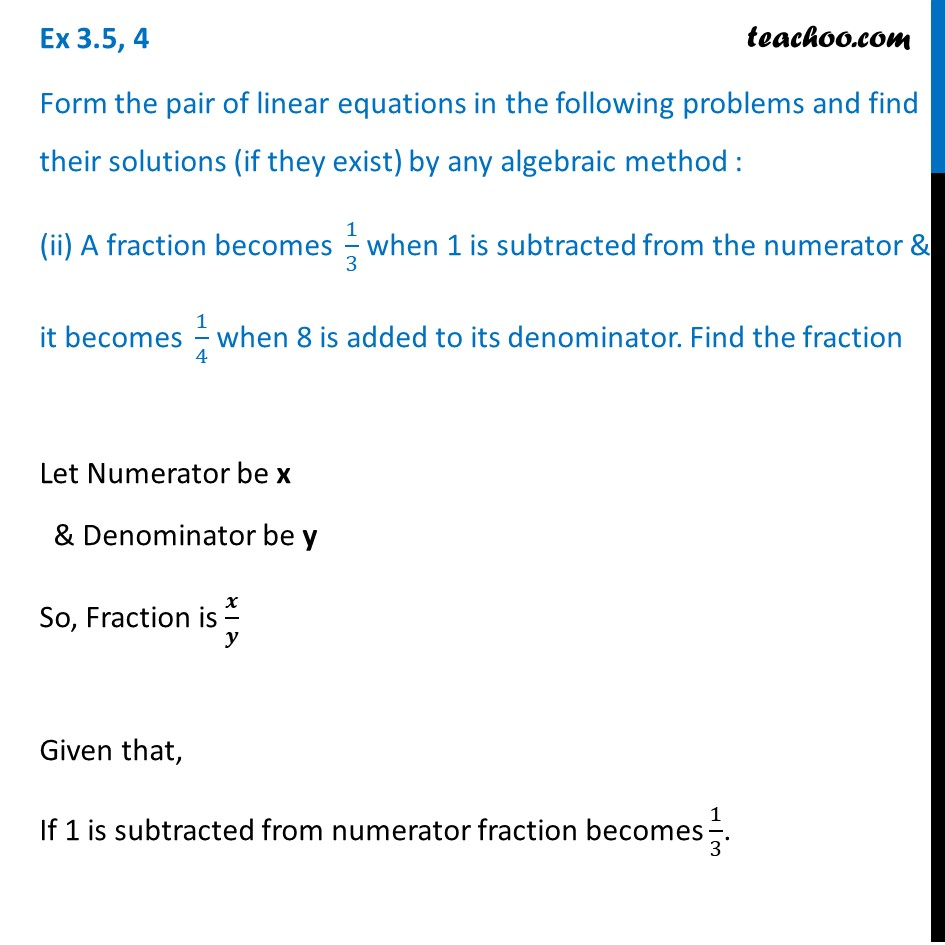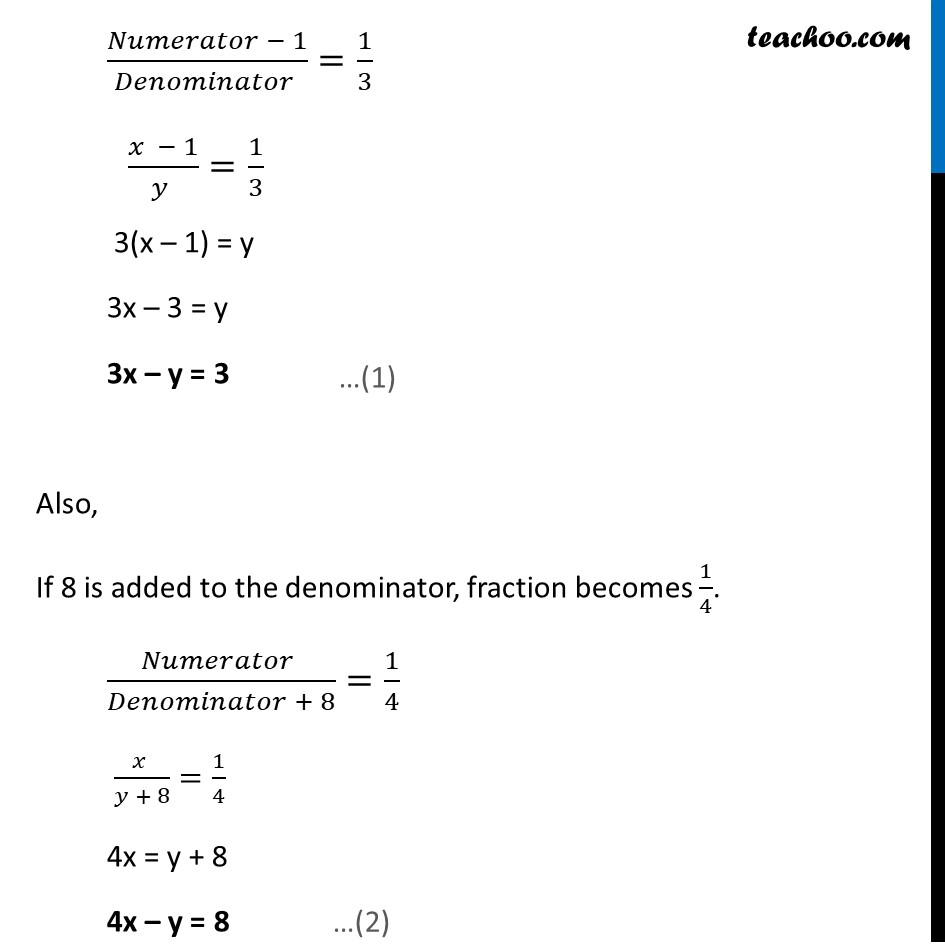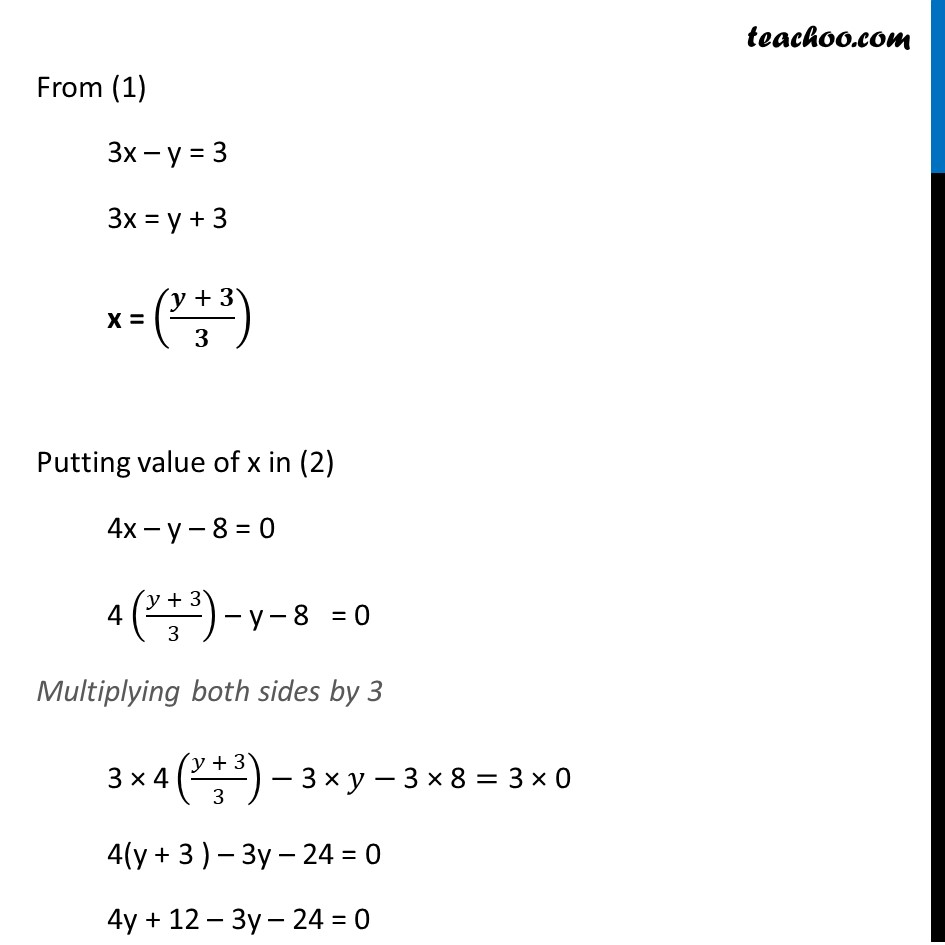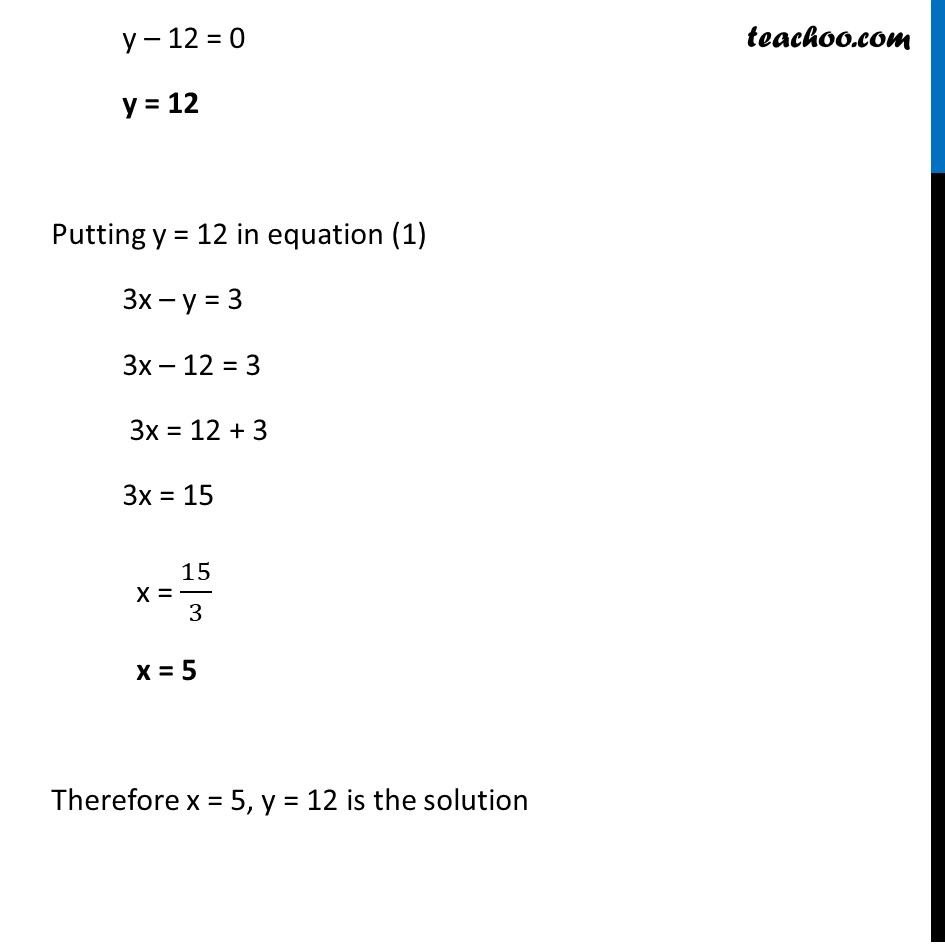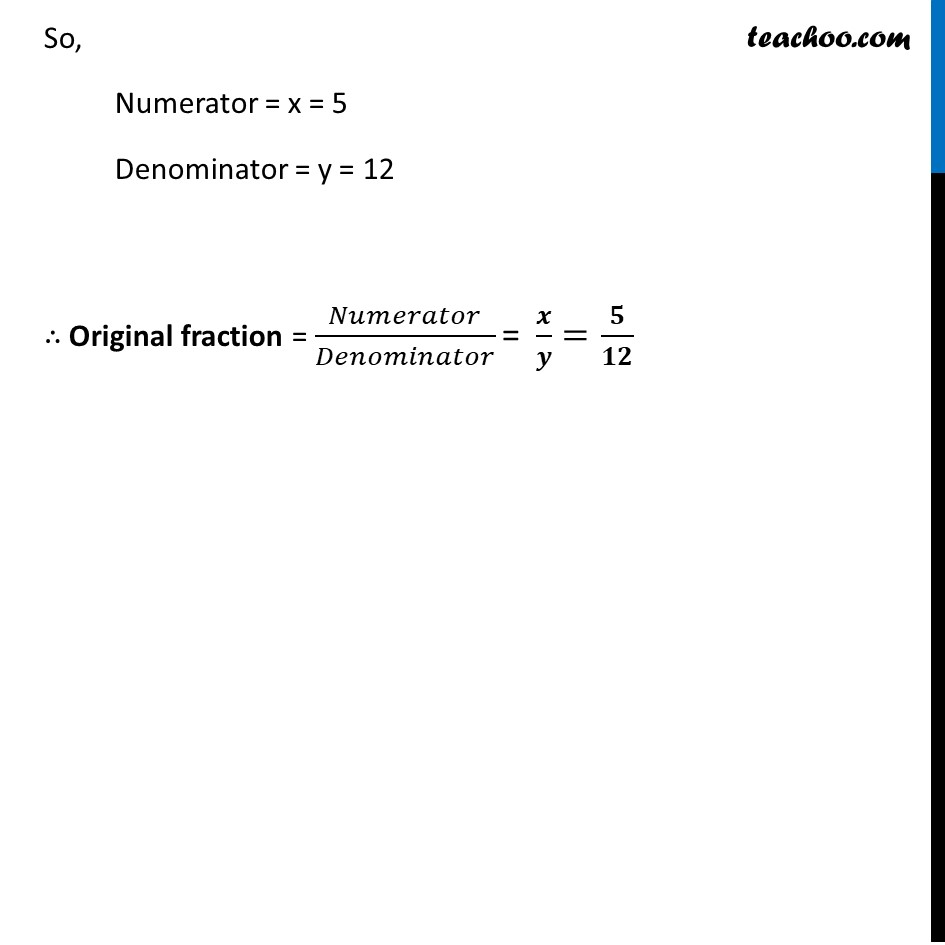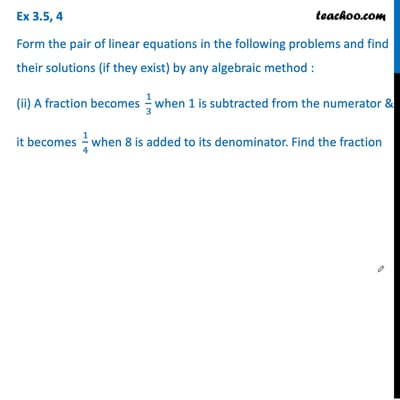This video is only available for Teachoo black users

### Transcript

Ex 3.5, 4 Form the pair of linear equations in the following problems and find their solutions (if they exist) by any algebraic method : (ii) A fraction becomes 1/3 when 1 is subtracted from the numerator & it becomes 1/4 when 8 is added to its denominator. Find the fraction Let Numerator be x & Denominator be y So, Fraction is 𝒙/𝒚 Given that, If 1 is subtracted from numerator fraction becomes 1/3. (𝑁𝑢𝑚𝑒𝑟𝑎𝑡𝑜𝑟 − 1)/(𝐷𝑒𝑛𝑜𝑚𝑖𝑛𝑎𝑡𝑜𝑟 )=1/3 (𝑥 − 1)/(𝑦 )=1/3 3(x – 1) = y 3x – 3 = y 3x – y = 3 Also, If 8 is added to the denominator, fraction becomes 1/4. (𝑁𝑢𝑚𝑒𝑟𝑎𝑡𝑜𝑟 )/(𝐷𝑒𝑛𝑜𝑚𝑖𝑛𝑎𝑡𝑜𝑟 + 8)=1/4 (𝑥 )/(𝑦 + 8)=1/4 4x = y + 8 4x – y = 8 From (1) 3x – y = 3 3x = y + 3 x = ((𝒚 + 𝟑)/𝟑) Putting value of x in (2) 4x – y – 8 = 0 4 ((𝑦 + 3)/3) – y – 8 = 0 Multiplying both sides by 3 3 × "4" ((𝑦 + 3)/3)−"3 × " 𝑦−"3 × 8"="3 ×" 0 4(y + 3 ) – 3y – 24 = 0 4y + 12 – 3y – 24 = 0 y – 12 = 0 y = 12 Putting y = 12 in equation (1) 3x – y = 3 3x – 12 = 3 3x = 12 + 3 3x = 15 x = 15/3 x = 5 Therefore x = 5, y = 12 is the solution So, Numerator = x = 5 Denominator = y = 12 ∴ Original fraction = 𝑁𝑢𝑚𝑒𝑟𝑎𝑡𝑜𝑟/𝐷𝑒𝑛𝑜𝑚𝑖𝑛𝑎𝑡𝑜𝑟 "= " 𝒙/𝒚=𝟓/𝟏𝟐